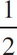# AP Physics 1 Question 82: Answer and Explanation

### Test Information

Question: 82

2. An object has a kinetic energy of k. If the speed of the object were doubled what would be the resulting kinetic energy?

• A.K
• B. K
• C. 2K
• D. 4K

DK =mv2, so if we double the velocity we don't double the kinetic energy, we actually quadruple it:
K' =m(2v)2 = 4 (mv2) = 4K